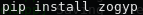# Image Subtraction

#### Image Subtraction and its challenges

Image subtraction is the process of taking two images, a new exposure of the night sky and a reference, and subtracting the reference from the new image. The purpose of this is to find changes in the sky without having to measure every star independantly.

There are three main challenges when doing real time image subtraction:

###### Alignment

When subtracting to images from eachother it is important that the stars in both frames occupy the same pixel space. This is vital, if the sources do not overlap they will leave a residual in the subtracted image that will look like a varying source.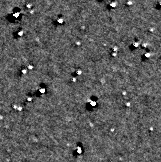Example of poor alignment

There are lots of ways to map one image onto another. One of the main ways is to use the World Coordinate System (WCS). The WCS is a grid that defines the coordinates of each spot in the image. By aligning the grids from each image, you in turn align both images. A good example of this is SWarp.

The Way ZiP does it is by finding sources is the image and building triangles. Using these triangles an affine transform is modelled. This is the rotation and stretches needed to allign the sources. Finally, a spline is used to modify warping of the edges. This is demonstrated in spalipy.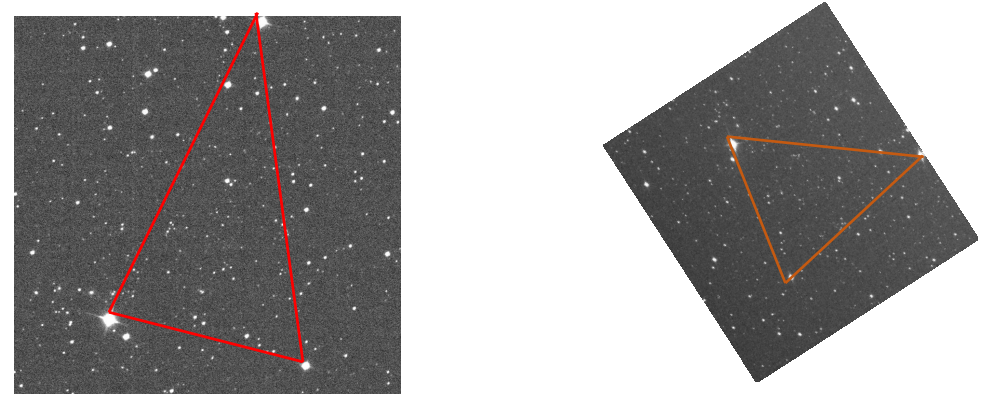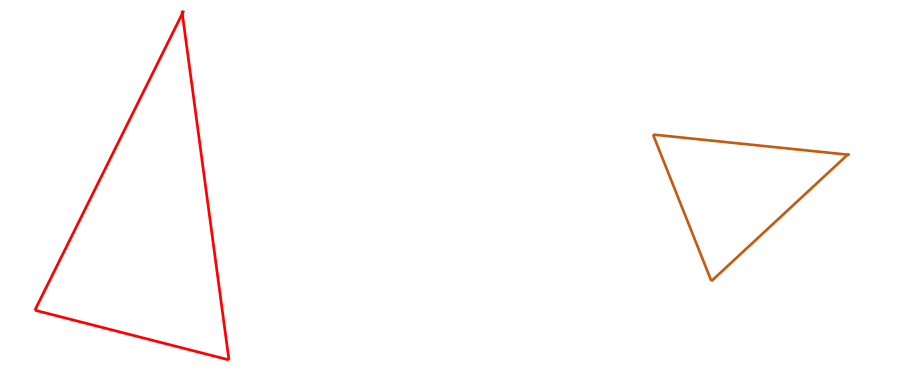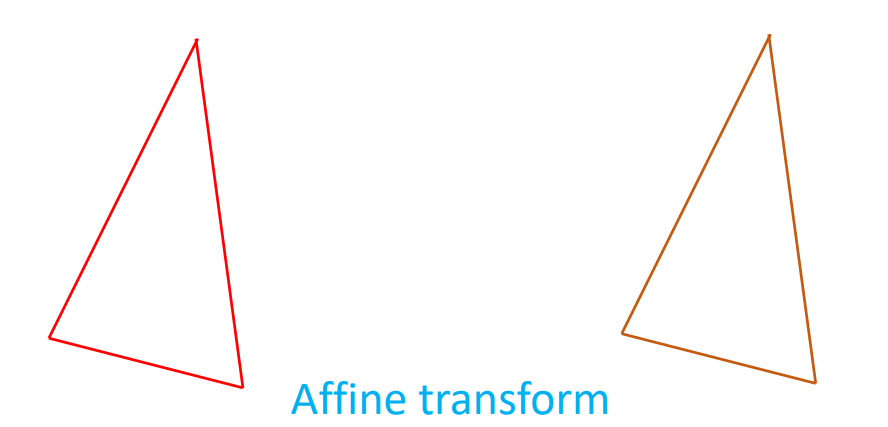Demonstration of an Affine transform on an image (except we use four stars)

###### PSF Fitting

A Point Spread Function (PSF) is the function associated with how elongated sources appear across the image. This is dependant on your optics and seeing conditions. A perfect PSF would mean each source occupies one pixel, this is pretty much impossible to achieve.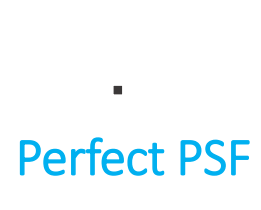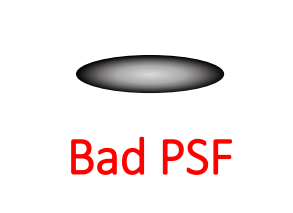Standards of PSF

We need to fit PSFs as different images can have different PSFs, which would result in a Halo around every source. An additional issue is introduced by having large fields of view, in that the PSF can vary dramaticaly across the field, making it difficult to estimate a good PSF.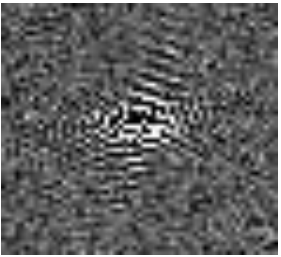Poor PSF fit

To model the PSF, ZiP uses PSFex. To overcome the issue of having a wide field of view, the images are cut into smaller sub images. The PSF is then modelled on each sub image instead. This also helps for computers with smaller amounts of RAM as the memory expense can be mitigated.

###### Processing time

Image subtraction is primarily used to find transients in the sky. Some transients are long lasting, however most last only a few weeks, or even days and evolve on relatively short timescales. Image subtraction can be a time consuming process, but we need to find transients quickly both to update our survey and to inform other telescopes of the transients location.

ZiP uses a two part parallelisation to speed up the subtraction. The first is hinted at above. By chopping a larger image into sub images and running the processing through these sub images simultaneously. The second is estimating the PSF of both images at the same time.Find the code on github

or with pip: